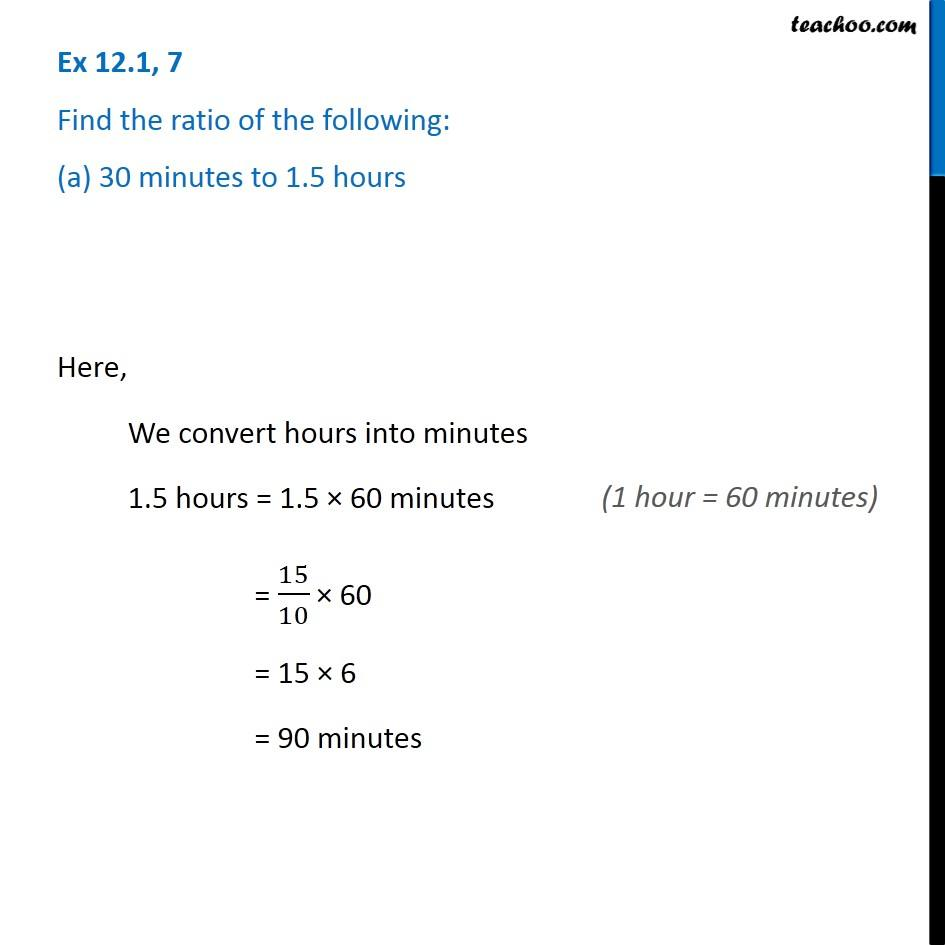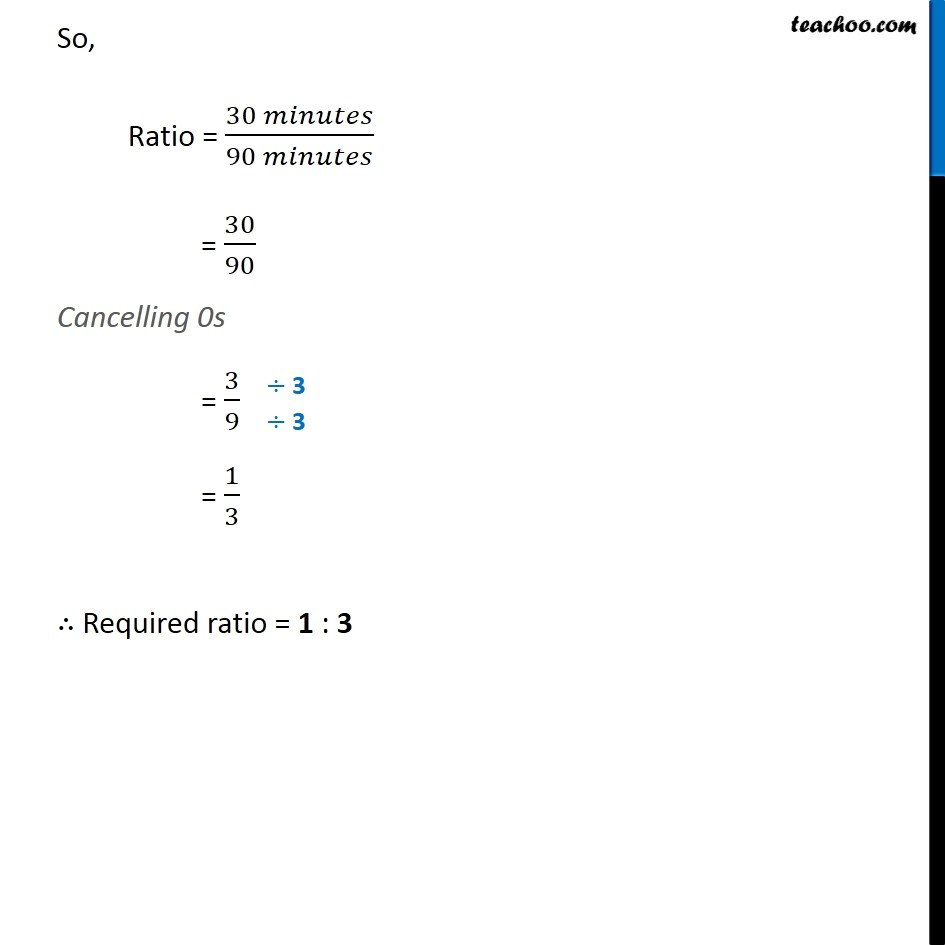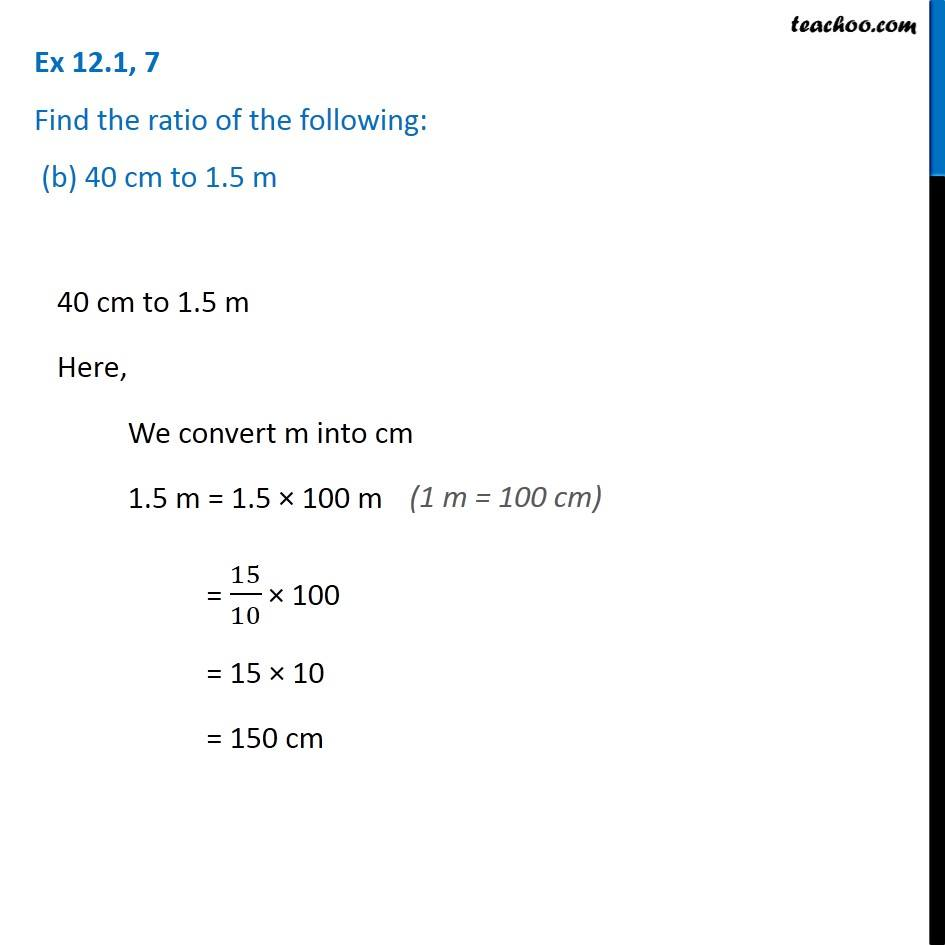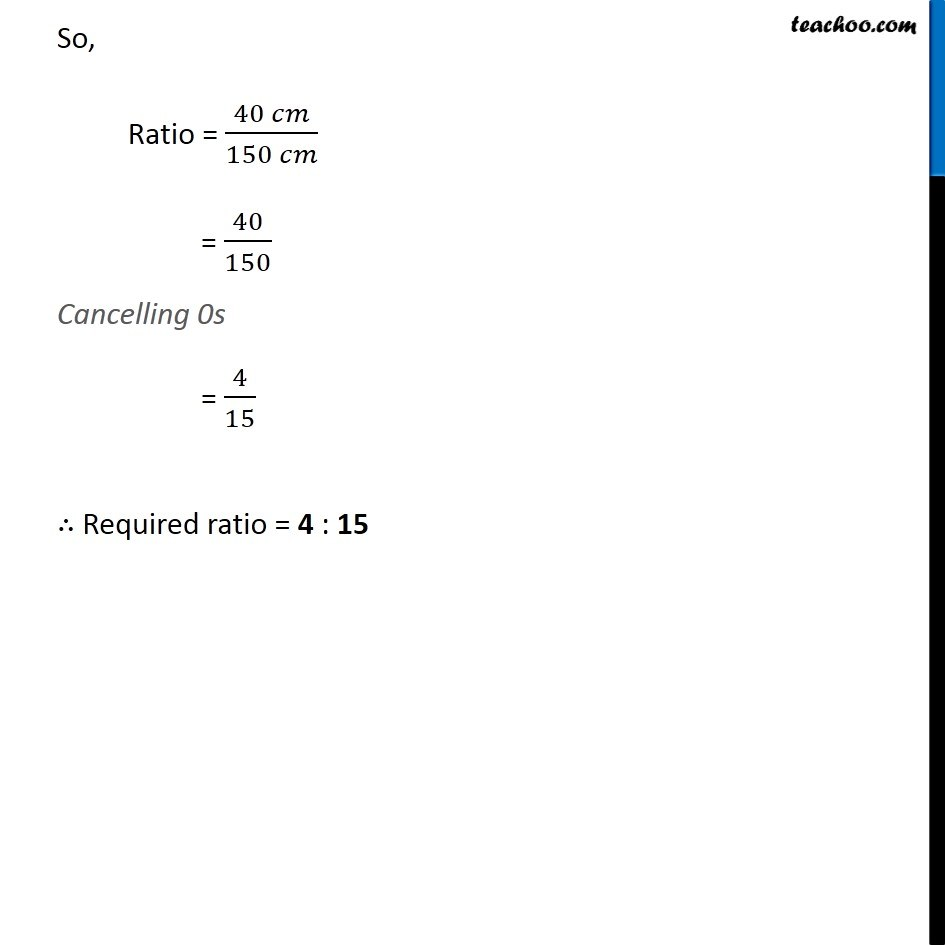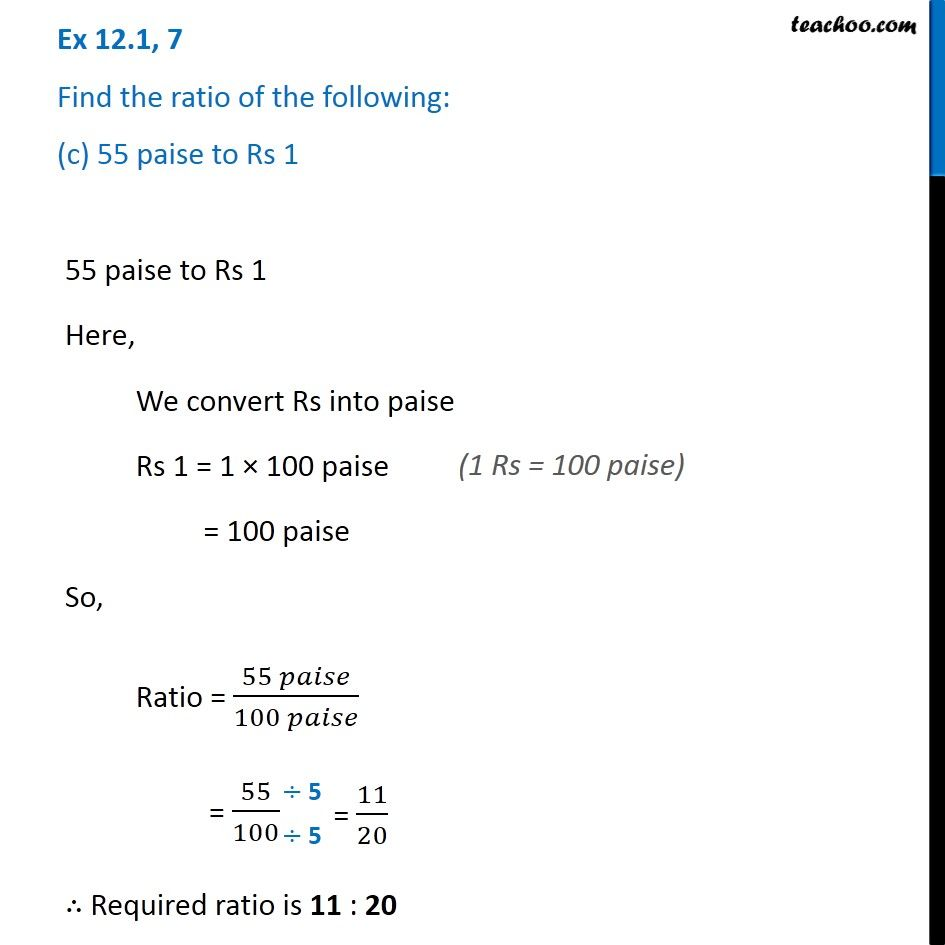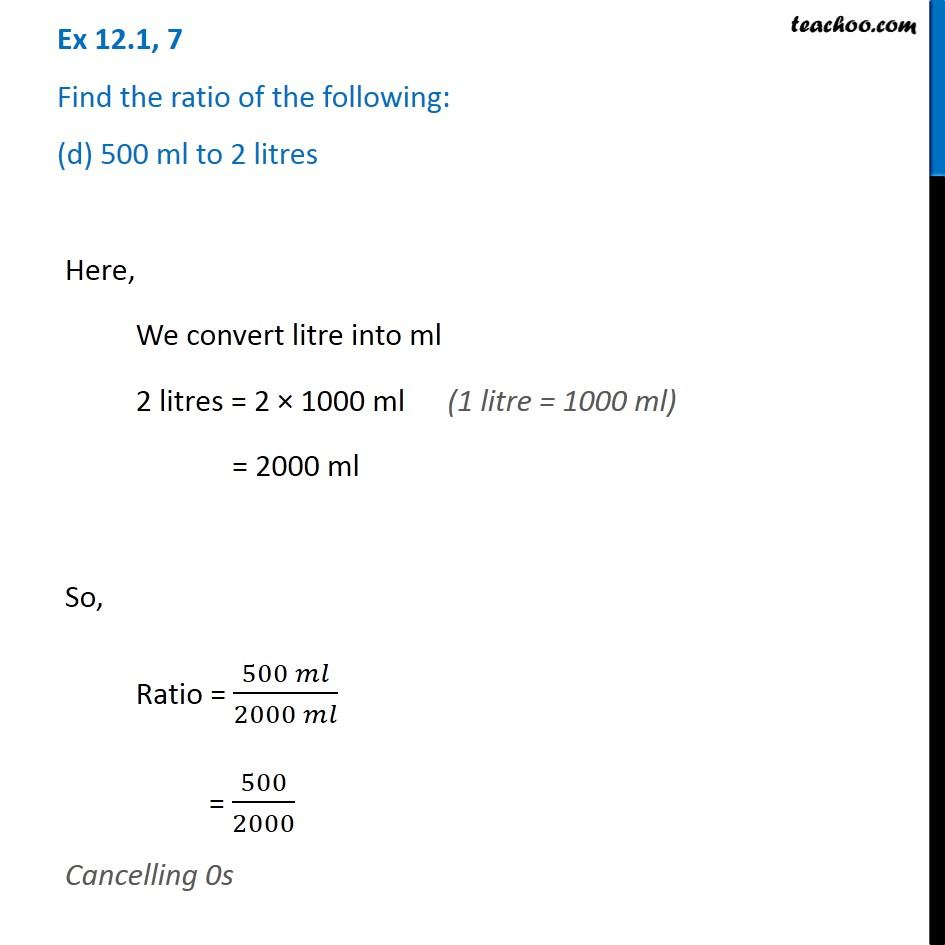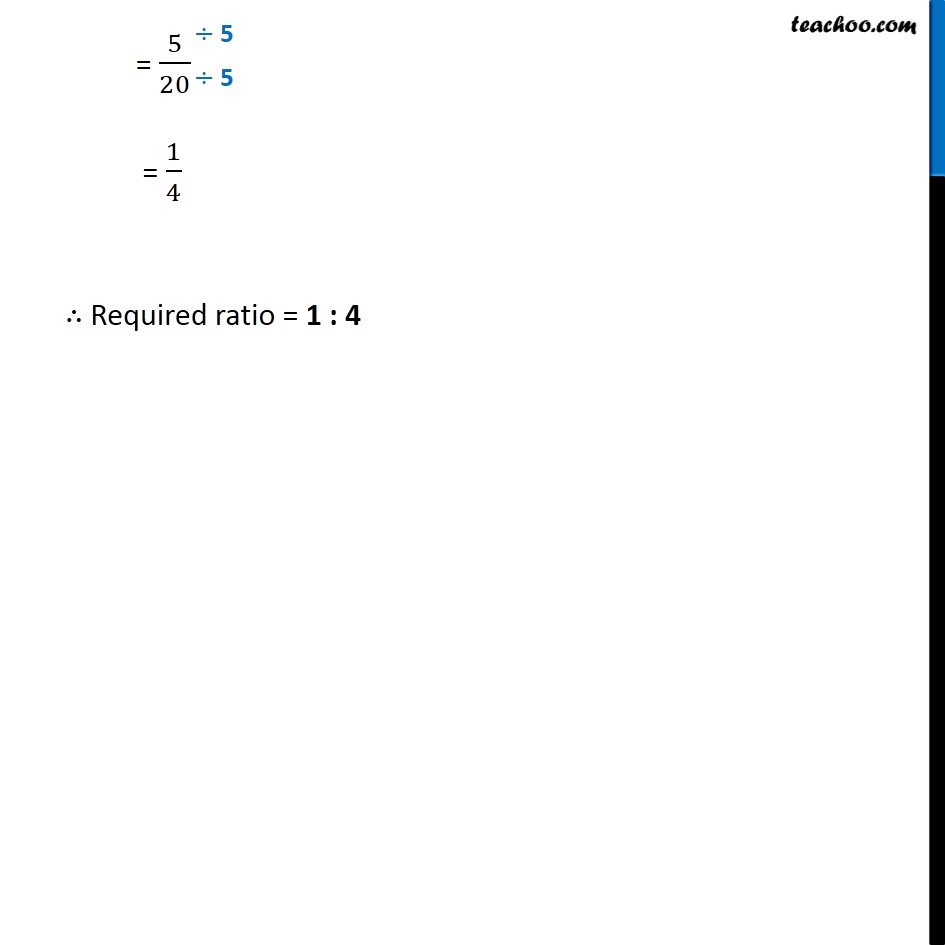Subscribe to our Youtube Channel - https://you.tube/teachoo

1. Chapter 12 Class 6 Ratio And Proportion
2. Serial order wise
3. Ex 12.1

Transcript

Ex 12.1, 7 Find the ratio of the following: (a) 30 minutes to 1.5 hours Here, We convert hours into minutes 1.5 hours = 1.5 × 60 minutes = 15/10 × 60 = 15 × 6 = 90 minutes So, Ratio = (30 𝑚𝑖𝑛𝑢𝑡𝑒𝑠)/(90 𝑚𝑖𝑛𝑢𝑡𝑒𝑠) = 30/90 Cancelling 0s = 3/9 = 1/3 ∴ Required ratio = 1 : 3 Ex 12.1, 7 Find the ratio of the following: (b) 40 cm to 1.5 m40 cm to 1.5 m Here, We convert m into cm 1.5 m = 1.5 × 100 m = 15/10 × 100 = 15 × 10 = 150 cm So, Ratio = (40 𝑐𝑚)/(150 𝑐𝑚) = 40/150 Cancelling 0s = 4/15 ∴ Required ratio = 4 : 15 Ex 12.1, 7 Find the ratio of the following: (c) 55 paise to Rs 1 55 paise to Rs 1 Here, We convert Rs into paise Rs 1 = 1 × 100 paise = 100 paise So, Ratio = (55 𝑝𝑎𝑖𝑠𝑒)/(100 𝑝𝑎𝑖𝑠𝑒) = 55/100 ∴ Required ratio is 11 : 20 Ex 12.1, 7 Find the ratio of the following: (d) 500 ml to 2 litresHere, We convert litre into ml 2 litres = 2 × 1000 ml = 2000 ml So, Ratio = (500 𝑚𝑙)/(2000 𝑚𝑙) = 500/2000 Cancelling 0s = 5/20 = 1/4 ∴ Required ratio = 1 : 4

Ex 12.1